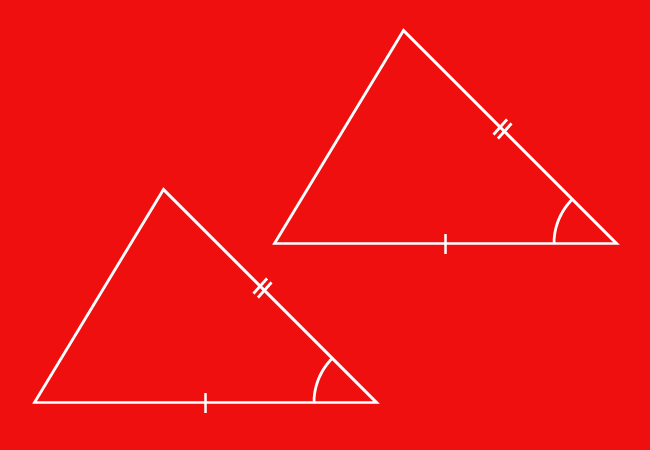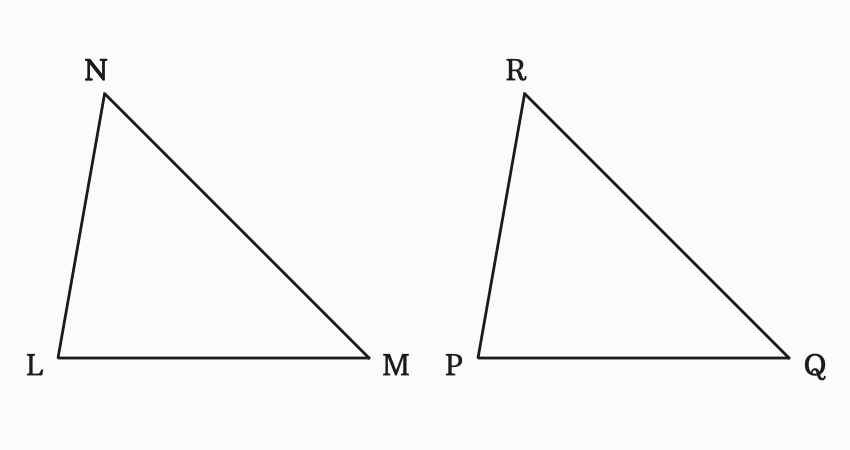# SAS Criterion for Congruence of Triangles

The triangles are congruent when the lengths of two sides and the included angle of one triangle are equal to the corresponding lengths of sides and the included angle of the other triangle. It is called Side-Angle-Side (SAS) criterion for the congruence of triangles.

## Introduction

In every triangle, there are three sides and three interior angles. The congruence of any two triangles can be determined by comparing the lengths of corresponding two sides and corresponding one included angle of them.If lengths of two sides and an angle between them of one triangle are equal to the lengths of corresponding sides and an included corresponding angle of other triangle, then the two triangles are congruent geometrically. Hence, the two triangles are called the congruent triangles.

Here, the comparison of corresponding two sides and corresponding the included angle of both triangles is a criteria for determining the congruence of any two triangles. Hence, it is called side-angle-side criterion and it is simply called SAS criterion for congruence of triangles.

The SAS (Side-Angle-Side) criterion can be studied in detail from an understandable example.

### Example

$\Delta LMN$ and $\Delta PQR$ are two triangles but their lengths and angles are unknown. However, the length of each side and the included angle can be measured by a ruler and a protractor respectively.In this case, measure any two sides and the angle between both sides in each triangle. It is measured that

In $\Delta ABC$, $LM \,=\, 5\,cm$, $MN \,=\, 6\,cm$ and $\angle LMN \,=\, 45^°$

In $\Delta PQR$, $PQ \,=\, 5\,cm$, $QR \,=\, 6\,cm$ and $\angle PQR \,=\, 45^°$

Compare the lengths of corresponding sides and the included angle of both triangles.

$(1).\,\,\,$ $LM \,=\, PQ \,=\, 5\,cm$

$(2).\,\,\,$ $MN \,=\, QR \,=\, 6\,cm$

$(3).\,\,\,$ $\angle LMN \,=\, \angle PQR \,=\, 45^°$

The lengths of two sides and the included angle of $\Delta ABC$ are exactly equal to the lengths of corresponding sides and the included angle of $\Delta PQR$. Hence, the two triangles are called the congruent triangle.

$\therefore \,\,\,\,\,\,$ $\Delta LMN \,\cong\, \Delta PQR$

The two corresponding sides and the included angle of both triangles are considered as a criteria in this example for checking the congruence of triangles. Therefore, the criteria is called SAS (Side-Angle-Side) criterion in geometry.

Latest Math Topics
Jun 26, 2023
Jun 23, 2023

###### Math Questions

The math problems with solutions to learn how to solve a problem.

Learn solutions

Practice now

###### Math Videos

The math videos tutorials with visual graphics to learn every concept.

Watch now

###### Subscribe us

Get the latest math updates from the Math Doubts by subscribing us.